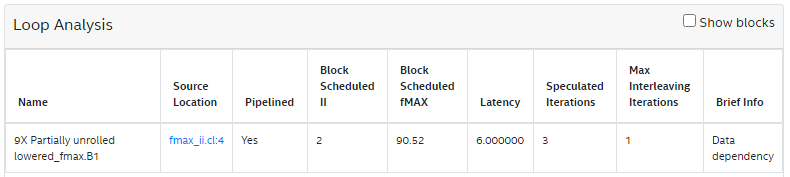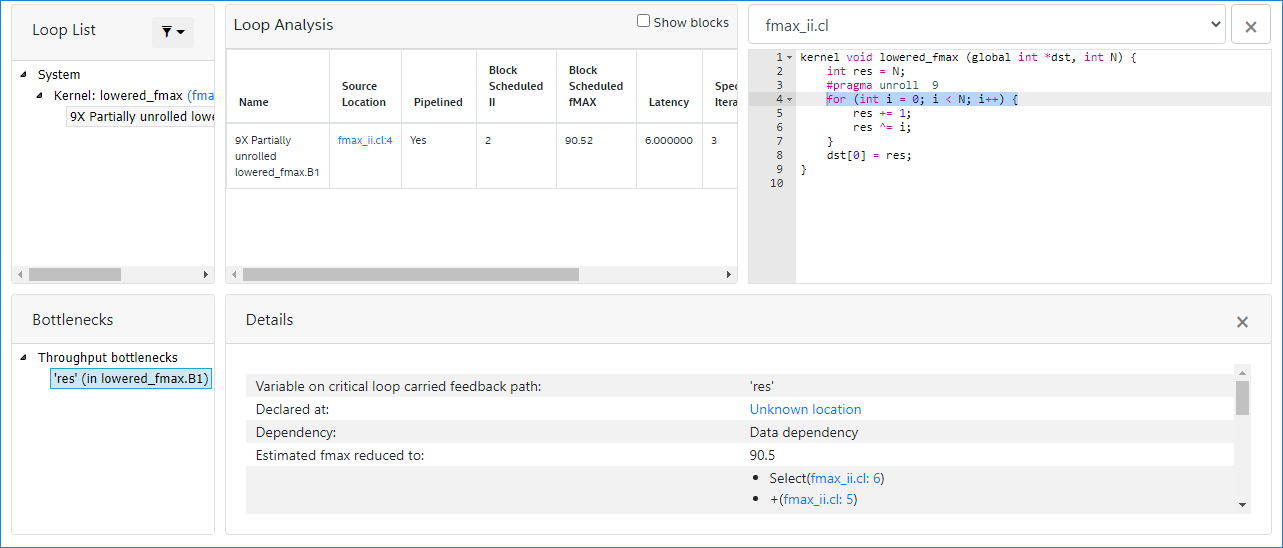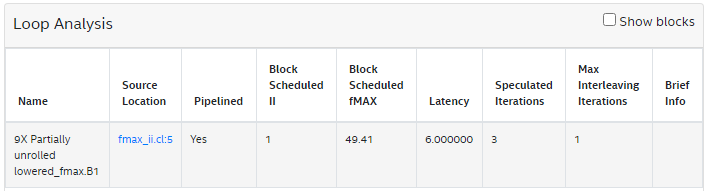ID 683521
Date 12/19/2022
Public

## 3.4.6. Loop Bottlenecks

Bottlenecks in a loop means one or more loop carried dependencies caused the compiler to reduce the number of data items to be processed concurrently (in the same clock cycle) or fMAX is reduced. Bottlenecks occur only on single work-item kernels and are always created for loops.

Before analyzing the throughput of a simple loop, it is important to understand the concept of dynamic initiation interval. The initiation interval (II) is the statically determined number of cycles between successive iteration launches of a given loop invocation. However, the statically scheduled II may differ from the actual realized dynamic II when considering interleaving.

Note: Interleaving allows the iterations of more than one invocation of a loop to execute in parallel, provided that the static II of that loop is greater than 1. By default, the maximum amount of interleaving for a loop is equal to the static II of that loop.

In the presence of interleaving, the dynamic II of a loop can be approximated by the static II of the loop divided by the degree of interleaving, that is, by the number of concurrent invocations of the loop that are in flight.

### Simple Loop Example

In a simple loop, the maximum number of data items to be processed concurrently (also known as maximum concurrency) can be expressed as:

ConcurrencyMAX = (Block latency × Maximum interleaving iterations) / Initiation Interval

Consider the following simple loop:

1  kernel void lowered_fmax (global int *dst, int N) {
2    int res = N;
3    #pragma unroll  9
4    for (int i = 0; i < N; i++) {
5      res += 1;
6      res ^= i;
7    }
8    dst = res;
9  }

The Loop Analysis report displays the following for the simple loop:The for loop in line:4 has a latency of 6, maximum interleaving iterations of 1, and initiation interval of 2. So, the maximum concurrency is 3 (latency of 6 / II of 2). The bottleneck results from loop carried dependency caused by a data dependency on the variable res. This is reported in the Bottlenecks viewer as shown in the following image:Another type of loop carried dependency is memory dependency, as shown in the following example:

for (…)
for (…)
… = mem[x];
mem[y] = …;


### Nested Loop Example

In a nested loop, the maximum concurrency is more difficult to compute. For example, the loop carried dependency in a nested loop does not necessarily affect the initiation interval of the outer loop. Additionally, a nested loop often requires the knowledge of the inner loop's trip count. Consider the following example:

1  __kernel void serial_exe_sample( __global unsigned* restrict input,
2                                   __global unsigned* restrict output,
3                                    int N ) {
4    unsigned offsets;
5    unsigned size;
6    unsigned results;
7    for (unsigned i = 0; i < N; i++) {
8      offsets[i] = input[i];
9    }
10
11    for (unsigned i = 1; i < (N-1); i++) {
12      unsigned num =  offsets[i];
13      unsigned sum = 0;
14      // There's a memory dependency, so the inner loops are executed
15      // serially, i.e. the both loops finish before the next iteration
16      // of i in the outer loop can start.
17      for (unsigned j = 0; j < num; j++) {
18        size[j] = offsets[i+j] - offsets[i+j-1];
19      }
20      for (unsigned j = 0; j < 4; j++) {
21        results[j] = size[j];
22      }
23    }
24
25    // store it back
26    #pragma unroll 1
27    for (unsigned i = 0; i < 4; i++) {
28      output[i] = results[i];
29    }
30  }

In this example, the bottleneck is resulted from loop carried dependency caused by a memory dependency on the variable size. The size variable must finish loading in the loop in line:20 before the next outer loop (line:11) iteration can be launched. Therefore, the maximum concurrency of the outer loop is 1. This information is reported in the details sections of the Loop Analysis and Schedule Viewer reports.

After restructuring, consider applying the following loop pragmas or attributes on arrays:

Consider the previous Simple Loop Example where the concurrency is 3 as the initiation interval is 2. Applying #pragma II 1, as shown in the following code snippet, comes at the expense of lowered predicted fMAX from 90MHz to 50MHz:

1  kernel void lowered_fmax (global int *dst, int N) {
2      int res = N;
3      #pragma unroll  9
4      #pragma ii 1
5      for (int i = 0; i < N; i++) {
6  	    res += 1;
7  	    res ^= i;
8      }
9      dst = res;
10  }